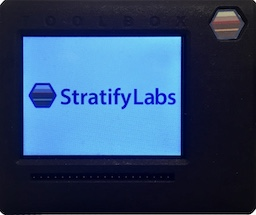# FFT on the ARM Cortex M3###### Introducing the Stratify Toolbox

Are you a printf()-er or a code-stepper? The Stratify Toolbox is a printf()-ing awesome debug tool!The ARM Cortex-M3 combined with a Fast Fourier Transform (FFT) implementation is a powerful, embedded digital signal processing (DSP) solution. The ARM Cortex-M3 is a mid-range microcontroller architecture with clock speeds over 100MHz and a powerful arithmetic logic unit (ALU). The FFT, a useful tool in many DSP applications, utilizes these features enabling the ARM Cortex-M3 to be a suitable replacement for dedicated DSP’s in some embedded applications.

## ARM Cortex-M3

The ARM Cortex-M3 architecture is a 32-bit microcontroller architecture core. The core is implemented by various manufacturers, including NXP, TI, STMicro, and Atmel, as microcontrollers that are competitively priced against 8/16 bit cores. It offers substantial performance improvements over 8/16 bit architectures including various features that enable signal processing algorithms such as single cycle 32-bit multiply and bit reversal instructions as well as separate data and instruction busses. The FFT makes frequent use of both the multiply and bit-reversal instructions as well as takes advantage of the separate busses.

## FFT

The FFT is an algorithm, or more accurately a family of algorithms, that implement the Discrete Fourier Transform (DFT) in a “fast” way–hence the name. The FFT re-uses calculations to provide faster computing times but sacrifices a small amount of accuracy in doing so. It turns out there are many uses for a fast approximation of the DFT.

## FFT Applications

The FFT is used frequently in both audio (and other one-dimensional signals) and image processing applications. The FFT allows a signal to be analyzed in the frequency domain rather than the discrete time domain. The following graph shows a signal in the time domain (top) as well as the frequency domain (middle and bottom).The above graphs use the following equation for the time-domain representation:

$$y_n = cos(2 \pi 770n) + cos(2 \pi 1366n)$$

This signal represents the number five using dual-tone multi-frequency encoding (DTMF)–that is, the sound you hear when you are on a phone call and press the number five. When looking at this signal in the time domain as compared to the frequency domain, it is much easier to decipher the tone looking at the frequency plot, hence the usefulness of the FFT.

In the frequency plot (middle above), there are four spikes. The spikes on the left represent the frequencies at 770Hz and 1366Hz (the bottom plot zooms in on these spikes). The spikes on the right are a mirror image of the aforementioned frequencies. In fact, the FFT always mirrors all frequencies below half the sampling frequency (see Nyquist Rate for more information).

Image processing also uses the FFT. Consider the images below. The one on the left is the original while the other has had an edge detection algorithm applied.Image from Wikpedia: Edge Detection

Edge detection is done by using an operation called convolution. A filter matrix is “convolved” with an image to produce the edge detected image. Because convolution is computationally intensive in the time domain, frequency domain multiplication can be used instead to reduced the number of operations performed. This means, rather than convolving, the following steps are taken:

• first the FFT is applied to the image and the filter,
• the image and the filter are multiplied,
• finally, an inverse FFT is applied to the result in order to get the edge detected image.

Despite being more steps, this approach actually uses much less computing power than convolution. However, convolution is much easier than an FFT to implement in code. The code sample below helps to bridge that gap.

## FFT Code

The code below is a snippet for performing an FFT. ARM provides a DSP library as part of the Cortex Microcontroller Software Inteface Standard (CMSIS).

typedef struct {
int32_t real;
int32_t imag;
} fft_i32_t;

void core_fft_i32(fft_context_i32_t * context, fft_i32_t * dest, fft_i32_t * src, int sign){
int n, k, m;
int half_N;
int k2;
int twiddle;
int twiddle_jump;
int twiddle_max;
int n_jump;
int n_start;
fft_i32_t tmp_product;
fft_i32_t twiddle_factor;
fft_i32_t tmp;

//copy the signal input to the tmp variable
for(n=0; n < context->N; n++){
m = bit_reversal(n,context->order);
dest[n] = src[m];
}

//This loop performs the FFT using the Cooley-Tukey algorithm
half_N = context->N >> 1;
for(k=0; k < context->order; k++){
n = 0;
k2 = (1<<k);

//Calculate the twiddle jump and max for the stage
twiddle_max = (1<<(context->order - 1));
twiddle_jump = (1<<(context->order - k - 1)); //equals 2^(kmax - k)

//Calculate the n jump for the stage
n_jump = k2<<1;
n_start = 0;

for(twiddle = 0; twiddle < twiddle_max; twiddle += twiddle_jump){

//twiddle value in the table (always < N/2)
twiddle_factor.real = context->twiddle_table[twiddle].real;
twiddle_factor.imag = context->twiddle_table[twiddle].imag * sign;

//This loop executes a single butterfly operation (size-2 DFT operation)
for(n=n_start; n < context->N; n+= n_jump){
m = n+k2;
//This is the butterfly code
tmp_product = fft_mult_i32(dest[m], twiddle_factor);  //multiply
tmp = scale_add_i32(dest[n], tmp_product, context->scale); //accumlate
dest[m] = scale_subtract_i32(dest[n], tmp_product, context->scale);
dest[n] = tmp;

}
n_start++;
}

}
}


## Conclusion

The combination of the ARM CM3 and the FFT allow developers to execute some signal processing algorithms previously reserved for dedicated DSP hardware. This is possible due to the single cycle multiply as well as bit reversal instructions available on the CM3 and the high speed CPU cores that are available. All of this functionality comes at power budgets and price points that are competitive with 8/16 microcontroller architectures.

X